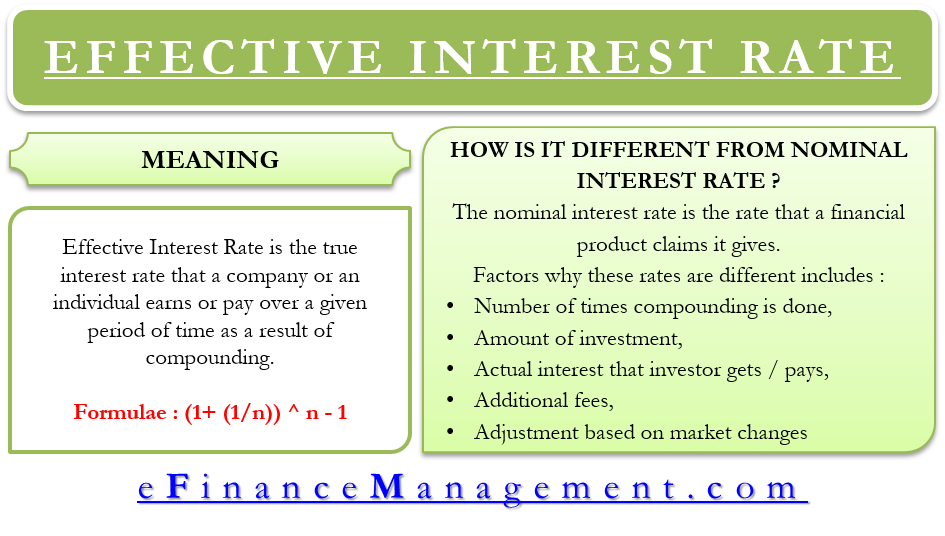Effective Interest Rate – Meaning, Formula, Importance And More

Effective Interest Rate is the true interest rate that a company or an individual earns or pay over a given period of time as a result of compounding. It could be an interest rate on investment, a loan or any other financial product.

One can also call such a rate as the effective rate, annual equivalent rate, discount rate, the internal rate of return, yield to maturity, market interest rate, required interest rate and the annual percentage rate (APR).

Effective interest rate is a crucial term in finance as it helps to compare varying financial products that calculate interest on a compounding basis. These financial products can be lines of credits, loans or investment instruments such as deposit certificates.

How it’s Different from Nominal Interest?

The nominal interest rate is the rate that a financial product claims it gives. For example, if a fixed deposit (FD) offers 8% interest, this 8% is the nominal interest rate. An effective rate may differ from the nominal rate due to several factors. These factors are:

• Number of times the compounding is done for that financial product.
• Amount that an investor pays.
• Actual interest that the investor pays or gets.

Apart from these, there are more factors that can impact the effective interest rate to an even bigger extent. These are:

Additional fees:  the product may require the investor or borrower to pay some hidden fees. Inclusion of such fees in the calculation may alter the effective rate.

Adjusting amount on the basis of market rate: if an investor believes that the market rate for a product is more or less than the nominal interest rate, then they can bid less or more than the face value of the debt. For instance, if the market rate is higher in case of the loan, then the borrower pays less for the debt. This results in a higher effective yield.

For example, assume a \$1000 bond pays 5% or \$50 per year till the lifetime of the bond. If after a year the market interest rate rises say 6%, then the 5% bond will lose some of its value because a similar \$1000 bond is giving 6% now. So, an investor will go for the 5% bond only if its effective rate is 6%, i.e., he or she will pay less than \$1000 for the same 5% bond now.Formula

One can calculate the effective rate by taking the nominal rate and then adjusting it for the number of compounding periods. The formula for calculating the effective interest rate using the nominal rate is

Effective Annual Interest = (1+ (1/n)) ^ n – 1

Here ‘i’ is the nominal interest rate and ‘n’ is the number of compounding periods.

It is a general rule that as the number of compounding periods increase, the effective annual interest rate also rises. For instance, quarterly compounding gives a higher return in comparison to the semi-annual compounding, while monthly compounding gives more return than the quarterly. Similarly, daily compounding produces a higher return than monthly.

Though the effective rate increases as the number of compounding period increases, there is a limit to this. Even if one tries to compound infinite times, i.e., every second or microsecond, the limit will be hit. For calculating the continuously compounding effective annual interest rate, we raise the number “e” to the power of the interest rate. The number e equals to 2.71828.

For example, for a 10% rate, the continuously compounding effective annual interest rate would be 10.517%. In comparison, the daily compounding is 10.516% while monthly is 10.471%.

Why it’s Useful?

To demonstrate the usefulness of the effective rate, lets us take an example. Suppose, John invests money in an investment (A) that pays him 10% compounded monthly, and an investment (B) that pays him 10.1% compounded semi-annually. From the outset, it seems investment B is more profitable, but calculating the effective rate will tell the real thing.

The effective interest rate for investment A is 10.47%, while for investment B is 10.36%. So, on the basis of interest rate, investment A is more profitable.

When Banks Use Don’t Use Effective Rate?

When you apply for a loan, the banks or other financial organizations use the nominal interest rate and not the effective rate. They do this, to make you believe that you are paying a less interest rate. For instance, a loan that the bank says is at 12%, but when compounded monthly, the effective rate will be 12.69%

On the other hand, when the banks are paying interest on deposit, they usually advertise the effective annual rate. The reason they do this is the same – effective rate in deposit looks more attractive than the nominal one.1–3

1.
Chen J. What the Effective Annual Interest Rate Tells Us. Investopedia. August 2019. [Source]
2.
How to calculate the effective interest rate —  AccountingTools. AccountingTools. August 2019. [Source]
3.
Effective Annual Rate (EAR) – How to Calculate Effective Interest Rate. Corporate Finance Institute. August 2019. [Source]
Last updated on : September 2nd, 2019
What’s your view on this? Share it in comments below.